## Machine Learning

Certification Training
17974 Learners
6 Chapters +

# Regression - Machine Learning

This is the ‘Regression’ tutorial and is part of the Machine Learning course offered by Simplilearn. We will learn Regression and Types of Regression in this tutorial.

## Learning Objectives

Let us look at the objectives below covered in this Regression tutorial.

• Explain Regression and Types of Regression.
• Describe Linear Regression: Equations and Algorithms

## What is Regression?

In statistical modeling, regression analysis is a set of statistical processes for estimating the relationships among variables.

## Types of Regression

Let us look at the types of Regression below:

• Linear Regression
• Multiple Linear Regression
• Polynomial Regression
• Decision Tree Regression
• Random Forest Regression

### Linear Regression

Linear Regression is the statistical model used to predict the relationship between independent and dependent variables by examining two factors. The first one is which variables, in particular, are significant predictors of the outcome variable and the second one is how significant is the regression line to make predictions with the highest possible accuracy.

Linear Regression Formula

Linear regression is a linear approach for modeling the relationship between a scalar dependent variable y and an independent variable x.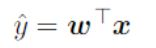where x, y, w are vectors of real numbers and w is a vector of weight parameters. The equation is also written as: y = wx + b, where b is the bias or the value of output for zero input.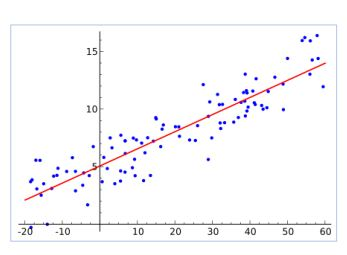Let's look at Linear Regression example:

### Profit Estimation of a Company

If you had to invest in a company, you would definitely like to know how much money you could expect to make. Let’s take a look at a venture capitalist firm and try to understand which companies they should invest in. First, we need to figure out:

• the companies to invest in.
• the profit the company makes.
• the company's expenses.

Now that we have our company’s data for different expenses, marketing, location and the kind of administration, we would like to calculate the profit based on all this different information.

Let's consider a single variable-R&D and find out which companies to invest in. We will now be plotting the profit based on the R&D expenditure and how much money they put into the research and development and then we will look at the profit that goes with that.

We have to draw a line through the data and when you look at that you can see how much they have invested in the R&D and how much profit it is going to make. We can also observe that the company that is spending more on R&D make good profits and thereby we invest in the ones that spend a higher rate in their R&D.

### Applications of Linear Regression

Few applications of Linear Regression mentioned below are:

• To determine the economic growth of a country or a state in the coming quarter.
• Can also be used to predict the GDP of a country.
• To predict what would be the price of a product in the future.
• To predict the number of runs a player will score in the coming matches.

### Multiple Linear Regression

It is a statistical technique used to predict the outcome of a response variable through several explanatory variables and model the relationships between them. It represents line fitment between multiple inputs and one output, typically:

y = w1x1 + w2x2 + b### Polynomial Regression

Polynomial regression is applied when data is not formed in a straight line. It is used to fit a linear model to non-linear data by creating new features from powers of non-linear features. Example: Quadratic features

x2 ’ = x2 2

y = w1x1 + w2x2 2 + 6 = w1x1 + w2x2 ’ + 6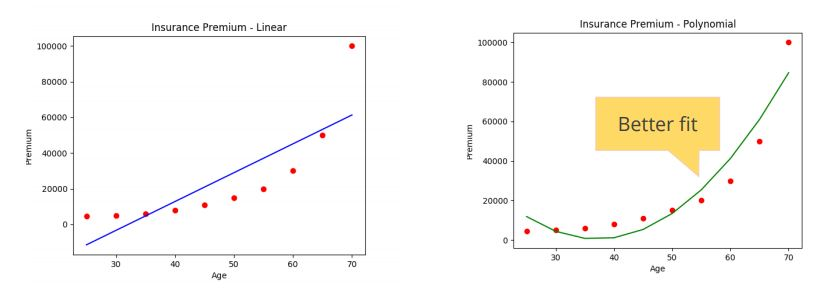Know more about Regression and its types. Click here!

## What is A Decision Tree?

A decision tree is a graphical representation of all the possible solutions to a decision based on a few conditions.

### Decision Tree Regression Algorithm

The algorithms involved in Decision Tree Regression are mentioned below.

• Consider data with two independent variables, X1 and X2.
• The algorithm splits data into two parts. Split boundaries are decided based on the reduction in leaf impurity.
• The algorithm keeps on splitting subsets of data till it finds that further split will not give any further value.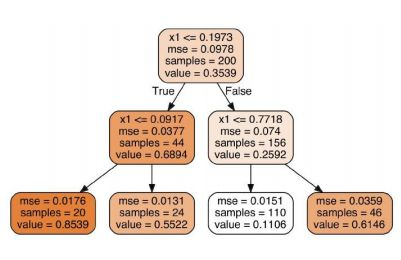• Calculate the average of dependent variables (y) of each leaf. That value represents the regression prediction of that leaf.
• This tree splits leaves based on x1 being lower than 0.1973. At second level, it splits based on x1 value again.
• At each node, the MSE (mean square error or the average distance of data samples from their mean) of all data samples in that node is calculated. The mean value for that node is provided as “value” attribute.

### Decision Tree Regression

Decision Trees can perform regression tasks. The following is a decision tree on a noisy quadratic dataset: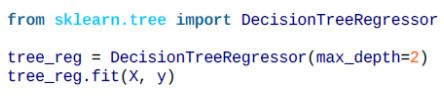## How Decision Trees Perform Regression

Let us look at the steps to perform Regression using Decision Trees.

• The dataset looks similar to classification DT. The main difference is that instead of predicting class, each node predicts value.
• This value represents the average target value of all the instances in this node.
• This prediction has an associated MSE or Mean Squared Error over the node instances.
• This mean value of the node is the predicted value for a new data instance that ends up in that node.

### Decision Tree Regression Plot

The regression plot is shown below. Notice that predicted value for each region is the average of the values of instances in that region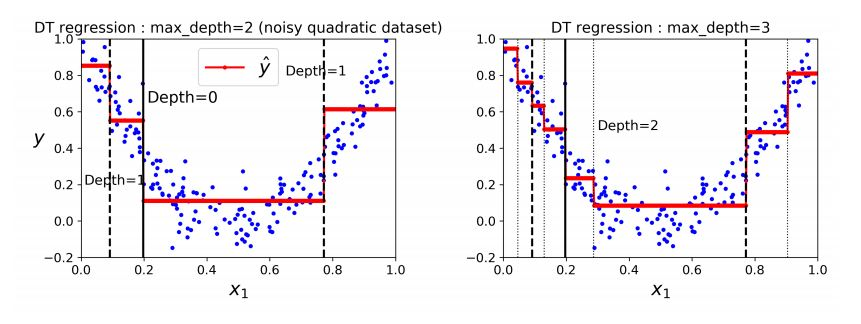## Decision Tree: Regularization

Let us understand Regularization in detail below.

• Decision Trees are non-parametric models, which means that the number of parameters is not determined prior to training. Such models will normally overfit data.
• In contrast, a parametric model (such as a linear model) has a predetermined number of parameters, thereby reducing its degrees of freedom. This, in turn, prevents overfitting.
• To prevent overfitting, one must restrict the degrees of freedom of a Decision Tree. This is called regularization.

## Decision Tree: Features of Regularization

Given below are some of the features of Regularization.

• Regularization is any modification made to the learning algorithm that reduces its generalization error but not its training error.
• In addition to varying the set of functions or the set of features possible for training an algorithm to achieve optimal capacity, one can resort to other ways to achieve regularization.## Decision Tree: Ways To Regularize Decision Trees

The table below explains some of the functions and their tasks.

 max_depth limit the maximum depth of the tree min_samples_split the minimum number of samples a node must have before it can be split Min_samples_leaf the minimum number of samples a leaf node must have Min_weight_fraction_leaf same as min_samples_leaf but expressed as a fraction of total instances max_leaf_nodes maximum number of leaf nodes max_features maximum number of features that are evaluated for splitting at each node

## Decision Tree: Regression (Cart Algorithm)

To achieve regression task, the CART algorithm follows the logic as in classification; however, instead of trying to minimize the leaf impurity, it tries to minimize the MSE or the mean square error, which represents the difference between observed and target output – (y-y’)2 ”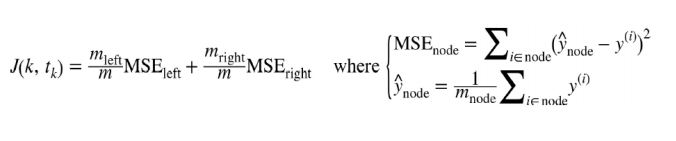J(k, tk ) represents the total loss function that one wishes to minimize. It is the sum of weighted (by a number of samples) MSE for the left and right node after the split.

## Decision Tree: Regression (Cart Algorithm) (Contd.)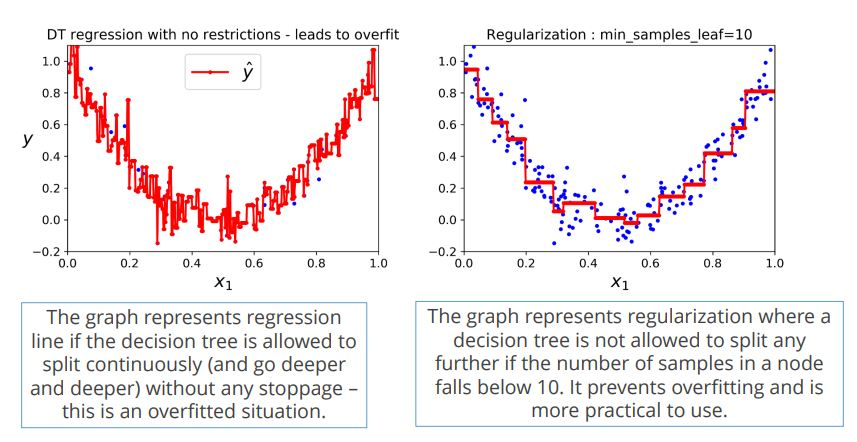## What is Random Forest?

Ensemble Learning uses the same algorithm multiple times or a group of different algorithms together to improve the prediction of a model. Random Forests use an ensemble of decision trees to perform regression tasks.

Random decision forest is a method that operates by constructing multiple decision trees, and the random forest chooses the decision of the majority of the trees as the final decision.

### Why Random Forest?

• No Overfitting
• Use of multiple trees reduce the risk of overfitting
• Training time is less
• High Accuracy
• Runs efficiently on a large database
• For large data, it produces highly accurate predictions.
• Estimates missing data
• Random forest can maintain accuracy when a significant proportion of the data is missing.

## Application of Random Forest

Let us look at the applications of Random Forest below:

Remote Sensing

Used in the ETM devices to look at images of the Earth's surface. The accuracy is higher and training time is less than many other machine learning tools.

Object Detection

Multi-class object detection is done using random forest algorithms and it provides a better detection in complicated environments.

Kinect

They are used as a random forest as part of the game, and it tracks the body movements along with it recreates the game.

## Random Forest Regression Algorithm

Let us look at the Algorithm steps for Random Forest below.

1. Pick any random K data points from the dataset

2. Build a decision tree from these K points

3. Choose the number of trees you want (N) and repeat steps 1 and 2

4. For a new data point, average the value of y predicted by all the N trees. This is the predicted value.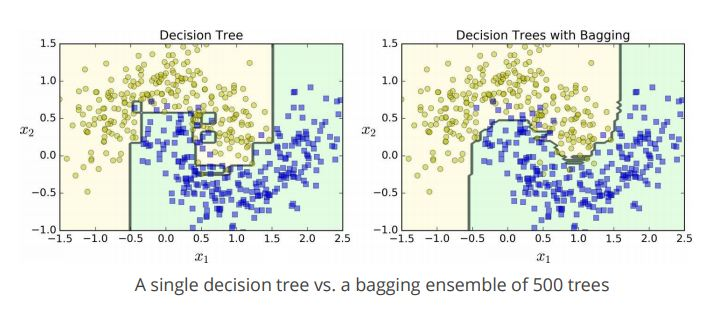## Mean Square Error (MSE)

Mean-squared error (MSE) is used to measure the performance of a model.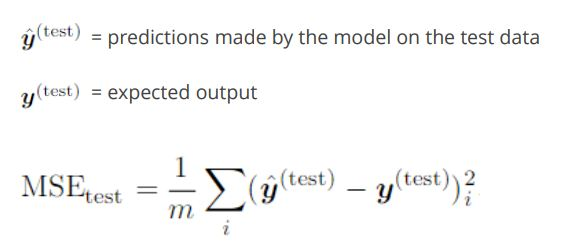• The objective is to design an algorithm that decreases the MSE by adjusting the weights w during the training session.

• The above function is also called the LOSS FUNCTION or the COST FUNCTION. The value needs to be minimized.

## Solving Linear Regression

Find parameters θ that minimize the least squares (OLS) equation, also called Loss Function:This decreases the difference between observed output [h(x)] and desired output [y].## Learning the Parameters

There are two ways to learn the parameters:

1. Normal Equation: Set the derivative (slope) of the Loss function to zero (this represents minimum error point).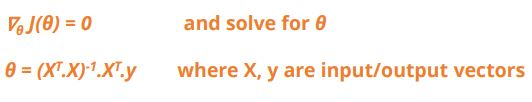1. LMS Algorithm: The minimization of the MSE loss function, in this case, is called LMS (least mean squared) rule or Widrow-Hoff learning rule. This typically uses the Gradient Descent algorithm.

## Deriving Normal Equation

To minimize MSEtrain, solve the areas where the gradient (or slope ) with respect to weight w is 0.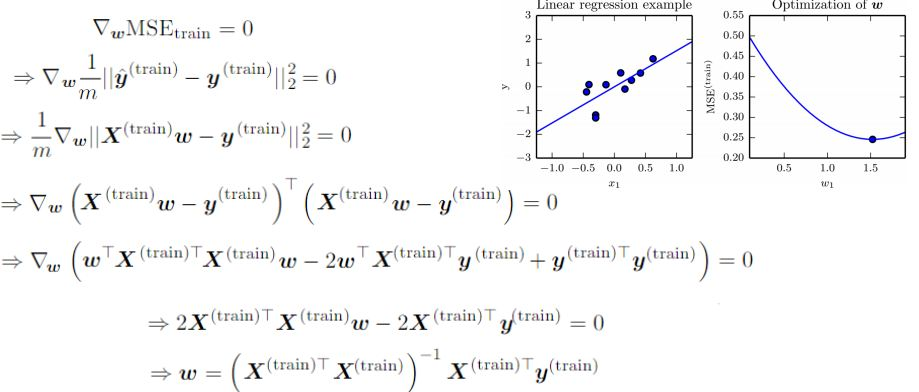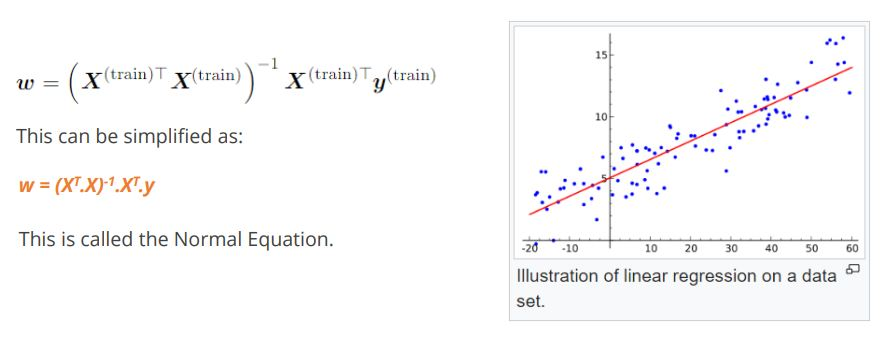This can be simplified as: w = (XT .X)-1 .XT .y This is called the Normal Equation.

## LMS Algorithm

In the case of Linear Regression, the hypotheses are represented as:Where θi ’s are parameters (or weights). θi ’s can also be represented as θ0*x0 where x0 = 1, so: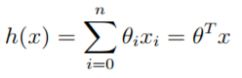The cost function (also called Ordinary Least Squares or OLS) defined is essentially MSE – the ½ is just to cancel out the 2 after derivative is taken and is less significant.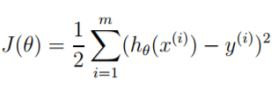It is advisable to start with random θ. Then repeatedly adjust θ to make J(θ) smaller.

## LMS Algorithm: Gradient Descent

Gradient descent is an algorithm used to minimize the loss function.

The J(θ) in dJ(θ)/dθ represents the cost function or error function that you wish to minimize, for example, OLS or (y-y')2.Minimizing this would mean that y' approaches y. In other words, observed output approaches the expected output. Other examples of loss or cost function include cross-entropy, that is, y*log(y’), which also tracks the difference between y and y‘.

## LMS Algorithm: Gradient Descent

Steps required to plot a graph are mentioned below.

1. The slope of J(θ) vs θ graph is dJ(θ)/dθ.
2. Adjust θ repeatedly. α is the learning rate.
3. This algorithm repeatedly takes a step toward the path of steepest descent.Calculate the derivative term for one training sample (x, y) to begin with.Update rule for one training sample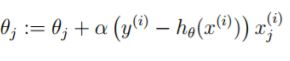## LMS Algorithm: Batch Gradient Descent

The algorithm moves from outward to inward to reach the minimum error point of the loss function bowl.

Extend the rule for more than one training sample: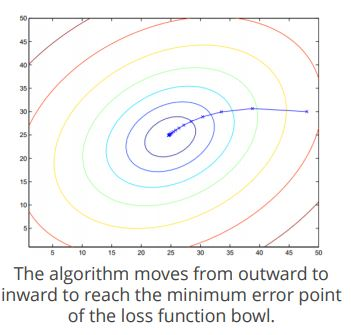• This method considers every training sample on every step and is called batch gradient descent.
• J is a convex quadratic function whose contours are shown in the figure.
• Gradient descent will converge to the global minimum, of which there is only one in this case.

## LMS Algorithm: Stochastic Gradient Descent

In this type of gradient descent, (also called incremental gradient descent), one updates the parameters after each training sample is processed.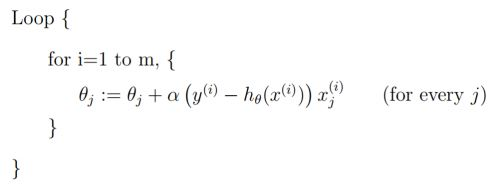• Unlike the batch gradient descent, the progress is made right away after each training sample is processed and applies to large data.
• Stochastic gradient descent offers the faster process to reach the minimum; It may or may not converge to the global minimum, but is mostly closed.

## LMS Algorithm: Gradient Descent Learning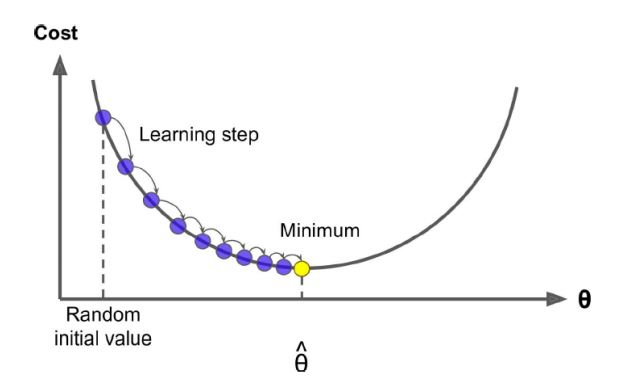The graph shows how the weight adjustment with each learning step brings down the cost or the loss function until it converges to a minimum cost.

An epoch refers to one pass of the model training loop.

## LMS Algorithm: Gradient Descent Learning Rate• Not all cost functions are good bowls. There may be holes, ridges, plateaus and other kinds of irregular terrain.
• In the figure, if random initialization of weights starts on the left, it will stop at a local minimum. If it starts on the right, it will be on a plateau, which will take a long time to converge to the global minimum.
• Fortunately, the MSE cost function for Linear Regression happens to be a convex function with a bowl with the global minimum.Get ahead with Machine Learning. Click for course description!

## Regularization

Let us understand Regularization in detail below.

• In addition to varying the set of functions or the set of features possible for training an algorithm to achieve optimal capacity, one can resort to other ways to achieve regularization.
• One such method is weight decay, which is added to the Cost function.• This approach not only minimizes the MSE (or mean-squared error), it also expresses the preference for the weights to have smaller squared L2 norm (that is, smaller weights).
• λ is a pre-set value. It influences the size of the weights allowed.
• This works well as smaller weights tend to cause less overfitting (of course, too small weights may cause underfitting).

## Regularizing a Model

Steps to Regularize a model are mentioned below.

• To regularize a model, a penalty (to the Cost function) called a Regularizer can be added: Ω(w)
• Hence the Cost function becomes:
• J(w) = MSEtrain + λ * Ω(w)
• In case of weight decay, this penalty is represented by: Ω(w) = wTw
• In essence, in the weight decay example, you expressed the preference for linear functions with smaller weights, and this was done by adding an extra term to minimize in the Cost function. Many other Regularizers are also possible.
• To summarize, the model capacity can be controlled by including/excluding members (that is, functions) from the hypothesis space and also by expressing preferences for one function over the other.

## Key Takeaways

Let us quickly go through what you have learned so far in this Regression tutorial.

• Regression is a Machine Learning technique to predict “how much” of something given a set of variables.
• The major types of regression are linear regression, polynomial regression, decision tree regression, and random forest regression.
• Regression uses labeled training data to learn the relation y = f(x) between input X and output Y. It works on linear or non-linear data.
• Gradient Descent is the most common technique used to train a regression model. It attempts to minimize the loss function to find ideal regression weights.
• Decision Trees are used for both classification and regression. For regression, Decision Trees calculate the mean value for each leaf node, and this is used as the prediction value during regression tasks.

## Conclusion

This concludes “Regression” tutorial. The next lesson is  "Classification."

### Find our Machine Learning Online Classroom training classes in top cities:

Name Date Place
Machine Learning 24 Apr -29 May 2021, Weekend batch Your City View Details
Machine Learning 3 May -21 May 2021, Weekdays batch San Francisco View Details
Machine Learning 7 May -11 Jun 2021, Weekdays batch New York City View Details
Related Courses
Learner Reviews
Related Articles
• Disclaimer
• PMP, PMI, PMBOK, CAPM, PgMP, PfMP, ACP, PBA, RMP, SP, and OPM3 are registered marks of the Project Management Institute, Inc.

#### Request more information

###### For business
Name*
Email*
Phone Number*
Your Message (Optional)
###### Our consultants will get in touch with you soon.

A Simplilearn representative will get back to you in one business day.

First Name*
Last Name*
Work Email*
Phone Number*
Company*
Job Title*